Next: Numerical instabilities Up: Integration of ODEs Previous: Euler's method

## Numerical errors

There are two major sources of error associated with a numerical integration scheme for o.d.e.s: namely, truncation error and round-off error. Truncation error arises in Euler's method because the curve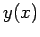is not generally a straight-line between the neighbouring grid-pointsand, as assumed above. The error associated with this approximation can easily be assessed by Taylor expandingabout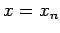: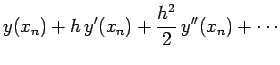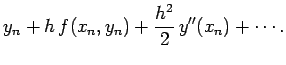(11)

A comparison of Eqs. (10) and (11) yields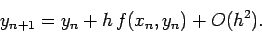(12)

In other words, every time we take a step using Euler's method we incur a truncation error of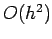, whereis the step-length. Suppose that we use Euler's method to integrate our o.d.e. over an-interval of order unity. This requires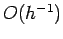steps. If each step incurs an error of, and the errors are simply cumulative (a fairly conservative assumption), then the net truncation error is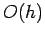. In other words, the error associated with integrating an o.d.e. over a finite interval using Euler's method is directly proportional to the step-length. Thus, if we want to keep the relative error in the integration below about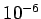then we would need to take about one million steps per unit interval in. Incidentally, Euler's method is termed a first-order integration method because the truncation error associated with integrating over a finite interval scales like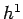. More generally, an integration method is conventionally called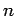th order if its truncation error per step is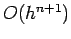.

Note that truncation error would be incurred even if computers performed floating-point arithmetic operations to infinite accuracy. Unfortunately, computers do not perform such operations to infinite accuracy. In fact, a computer is only capable of storing a floating-point number to a fixed number of decimal places. For every type of computer, there is a characteristic number,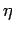, which is defined as the smallest number which when added to a number of order unity gives rise to a new number: i.e., a number which when taken away from the original number yields a non-zero result. Every floating-point operation incurs a round-off error of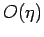which arises from the finite accuracy to which floating-point numbers are stored by the computer. Suppose that we use Euler's method to integrate our o.d.e. over an-interval of order unity. This entailsintegration steps, and, therefore,floating-point operations. If each floating-point operation incurs an error of, and the errors are simply cumulative, then the net round-off error is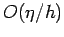.

The total error,, associated with integrating our o.d.e. over an-interval of order unity is (approximately) the sum of the truncation and round-off errors. Thus, for Euler's method,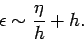(13)

Clearly, at large step-lengths the error is dominated by truncation error, whereas round-off error dominates at small step-lengths. The net error attains its minimum value,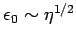, when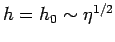. There is clearly no point in making the step-length,, any smaller than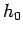, since this increases the number of floating-point operations but does not lead to an increase in the overall accuracy. It is also clear that the ultimate accuracy of Euler's method (or any other integration method) is determined by the accuracy,, to which floating-point numbers are stored on the computer performing the calculation.

The value ofdepends on how many bytes the computer hardware uses to store floating-point numbers. For IBM-PC clones, the appropriate value for double precision floating point numbers is(this value is specified in the system header file float.h). It follows that the minimum practical step-length for Euler's method on such a computer is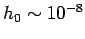, yielding a minimum relative integration error of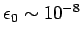. This level of accuracy is perfectly adequate for most scientific calculations. Note, however, that the correspondingvalue for single precision floating-point numbers is only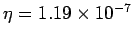, yielding a minimum practical step-length and a minimum relative error for Euler's method of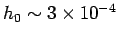and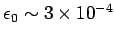, respectively. This level of accuracy is generally not adequate for scientific calculations, which explains why such calculations are invariably performed using double, rather than single, precision floating-point numbers on IBM-PC clones (and most other types of computer).Next: Numerical instabilities Up: Integration of ODEs Previous: Euler's method
Richard Fitzpatrick 2006-03-29